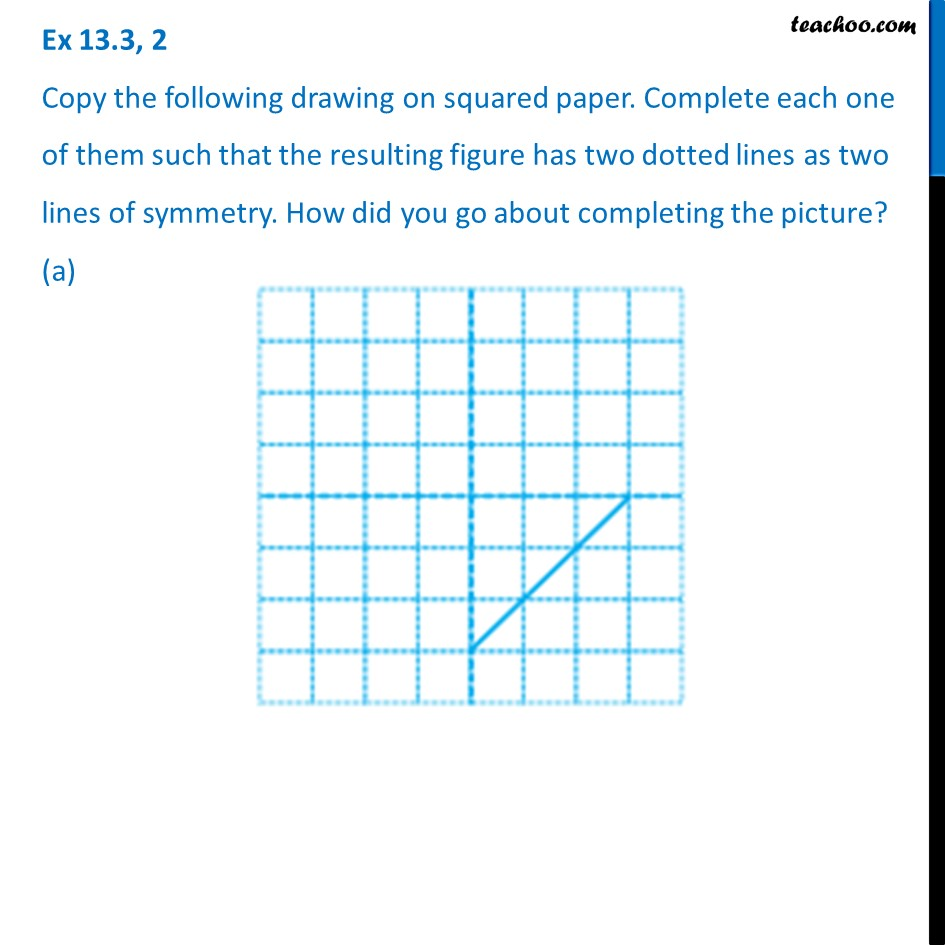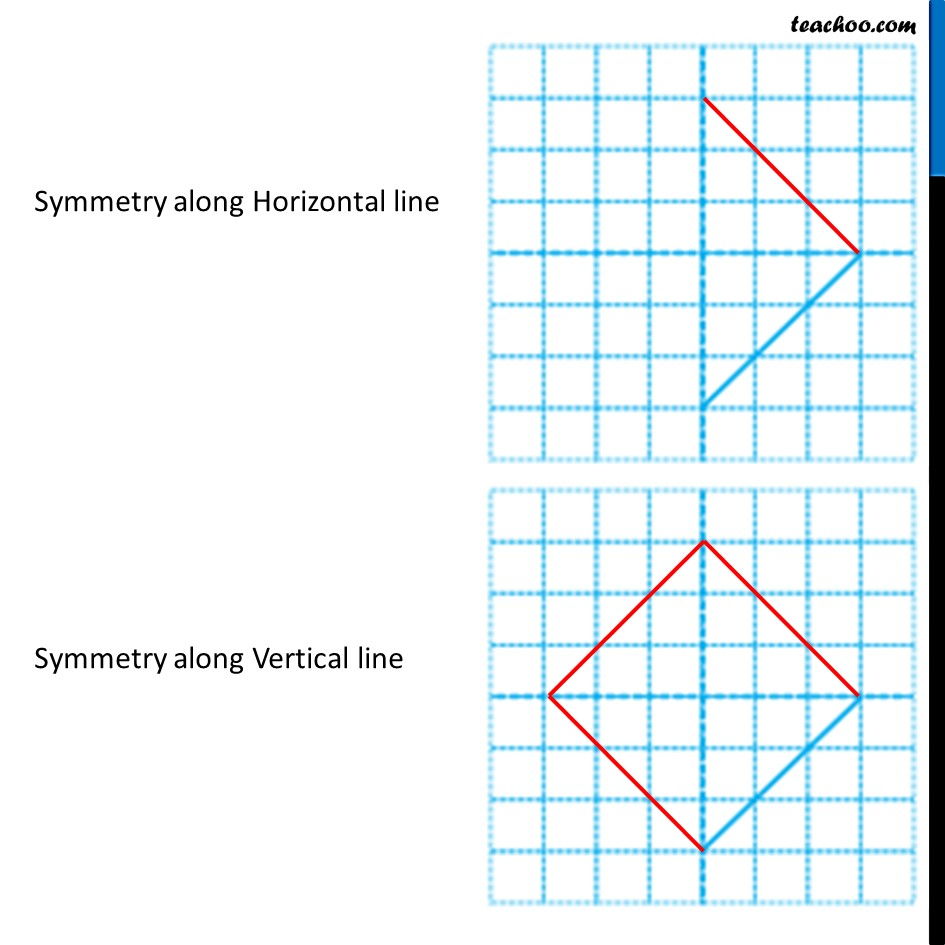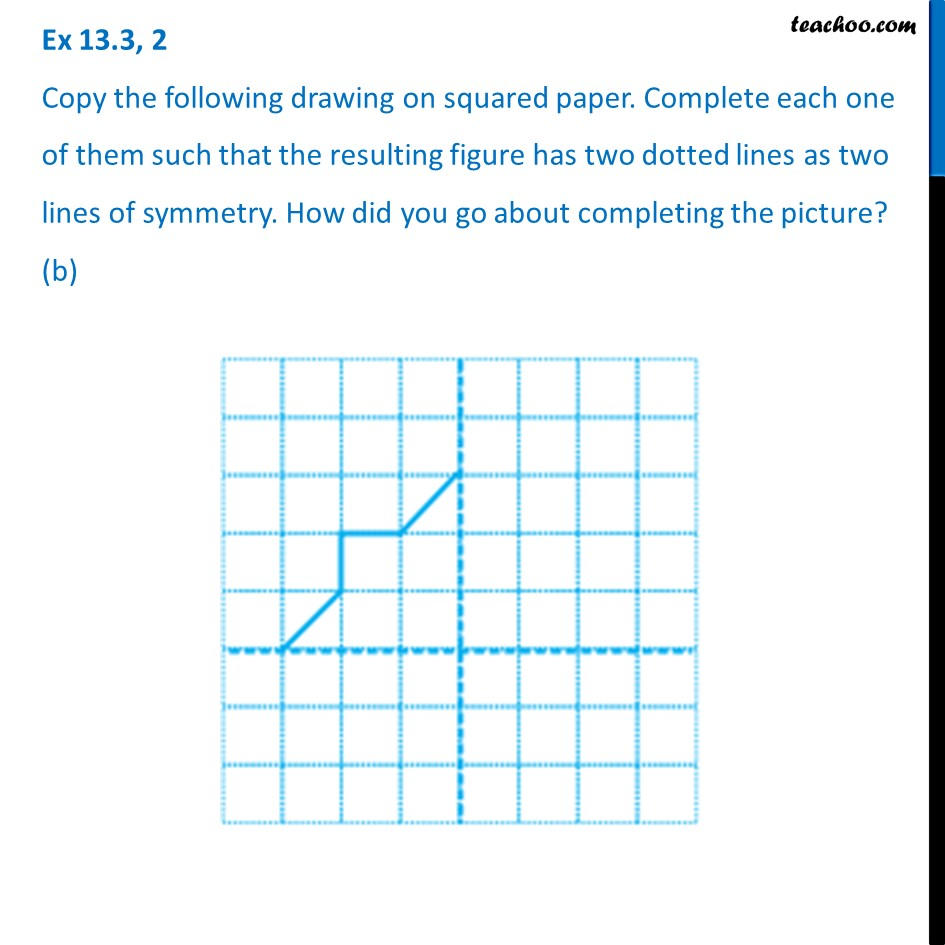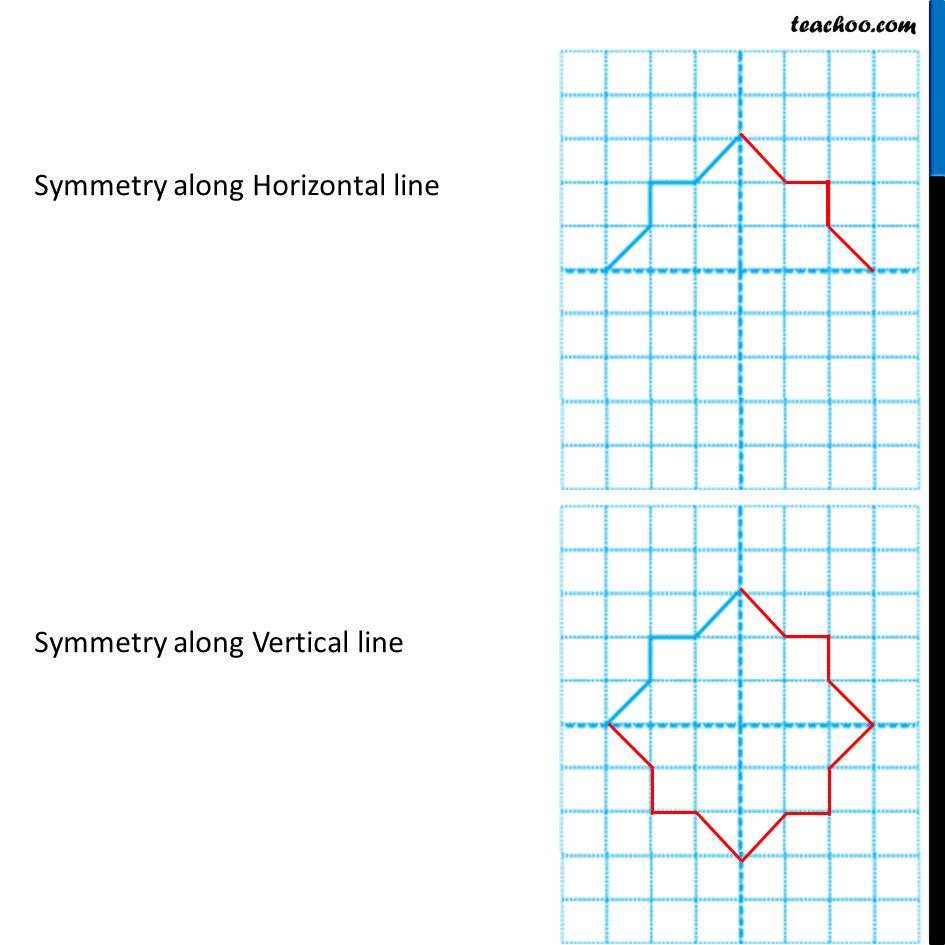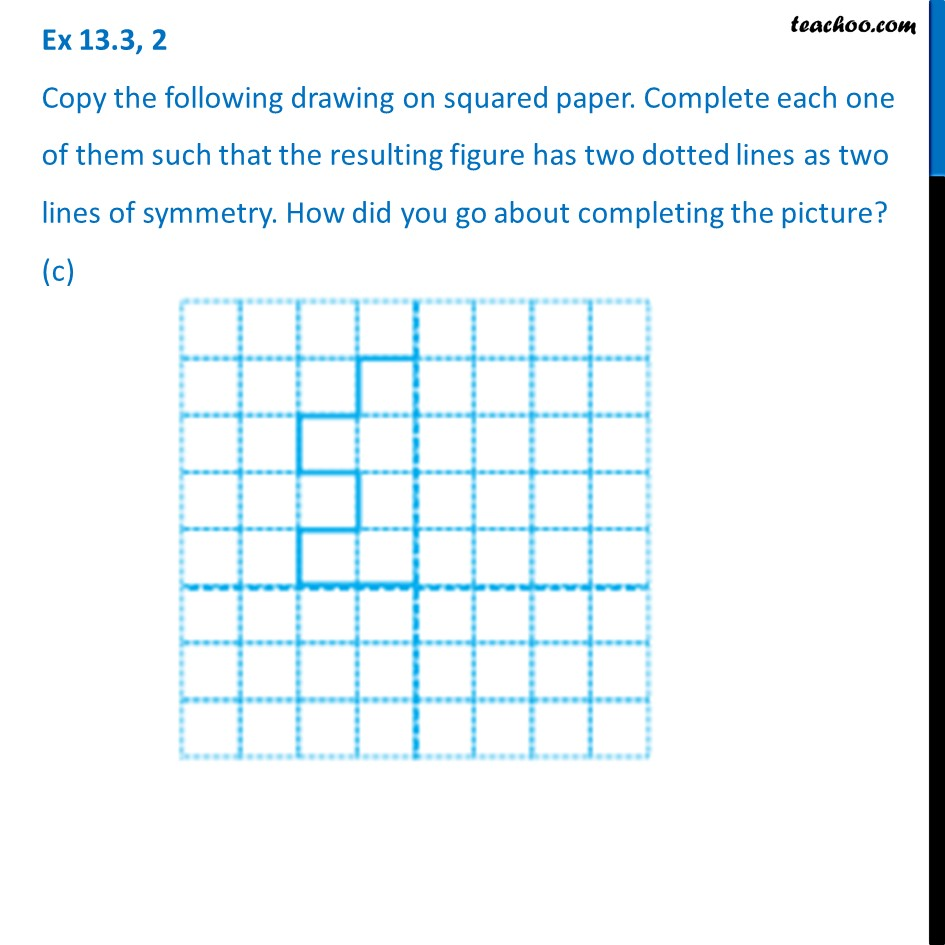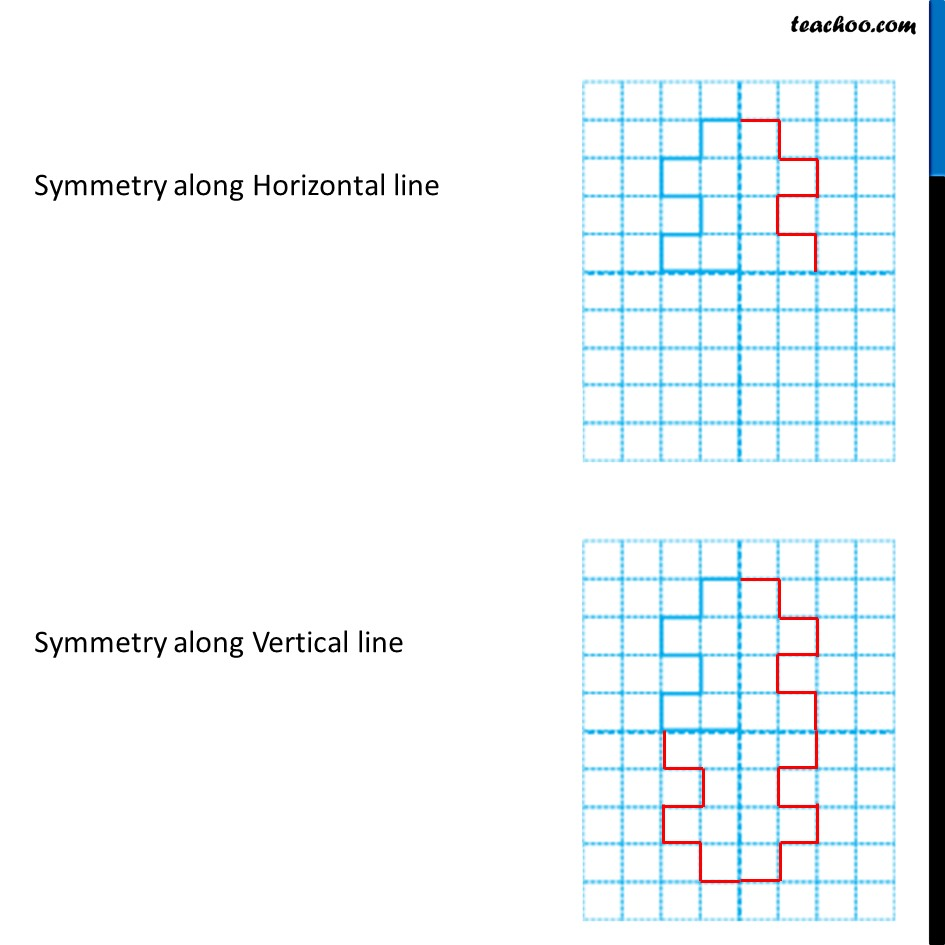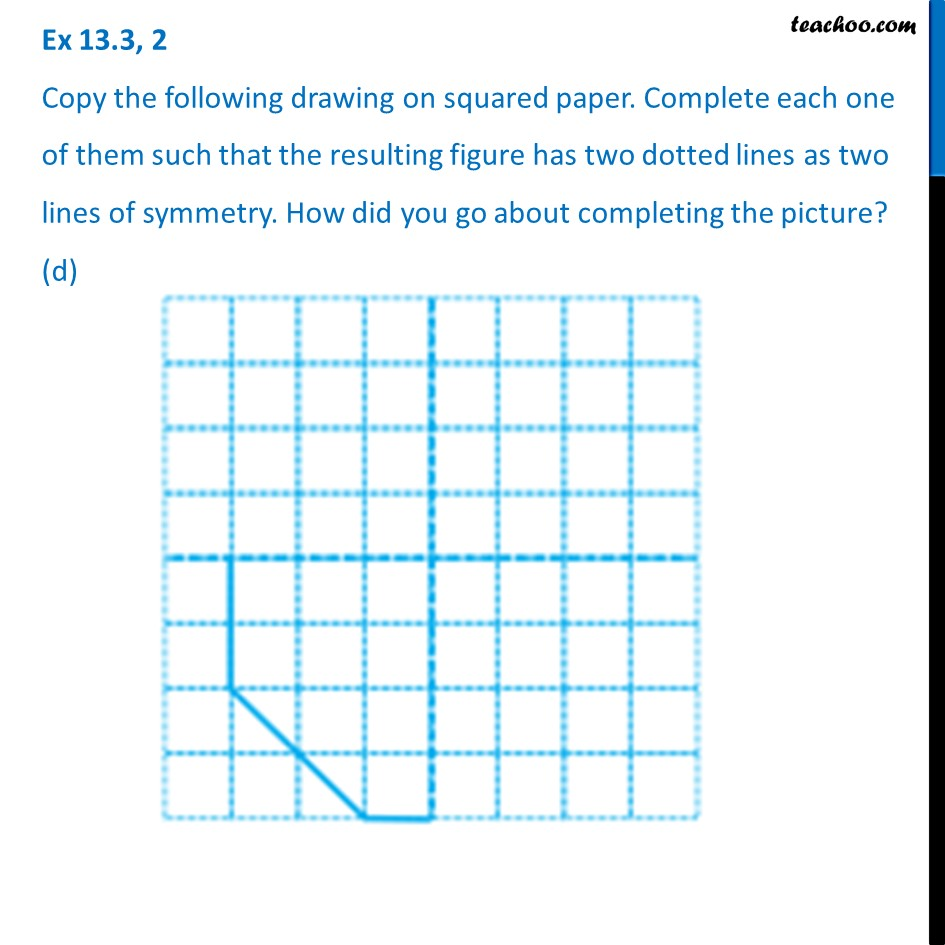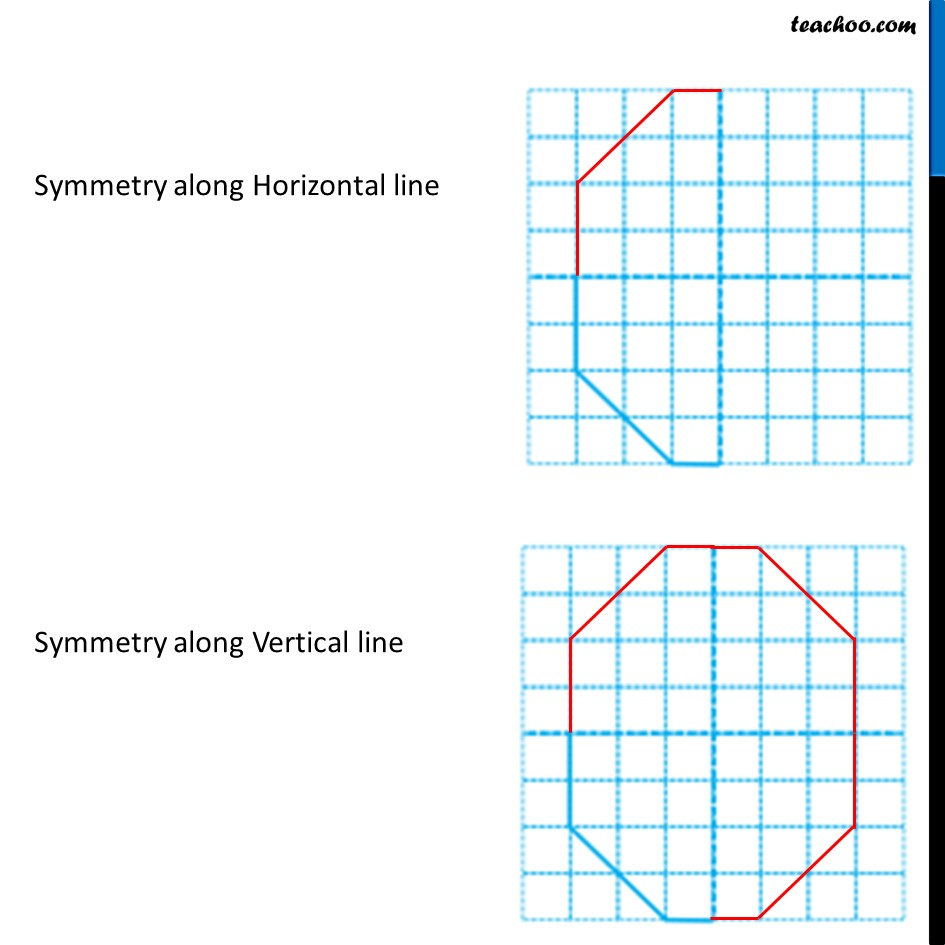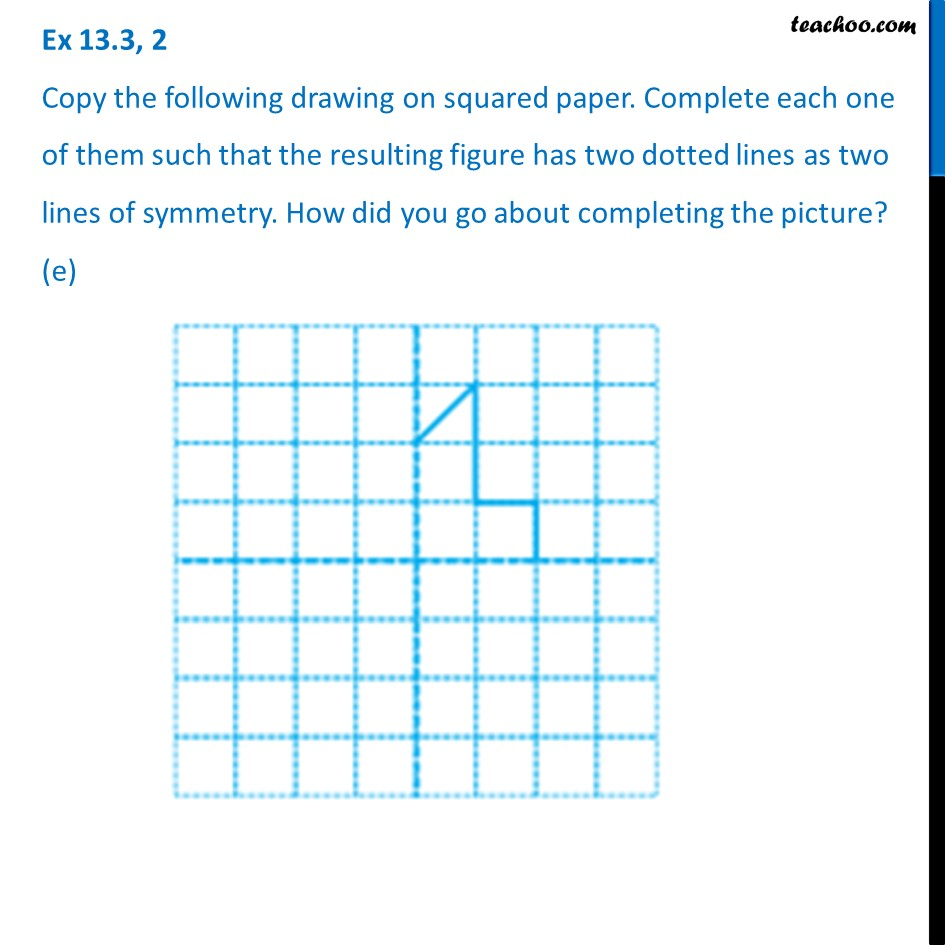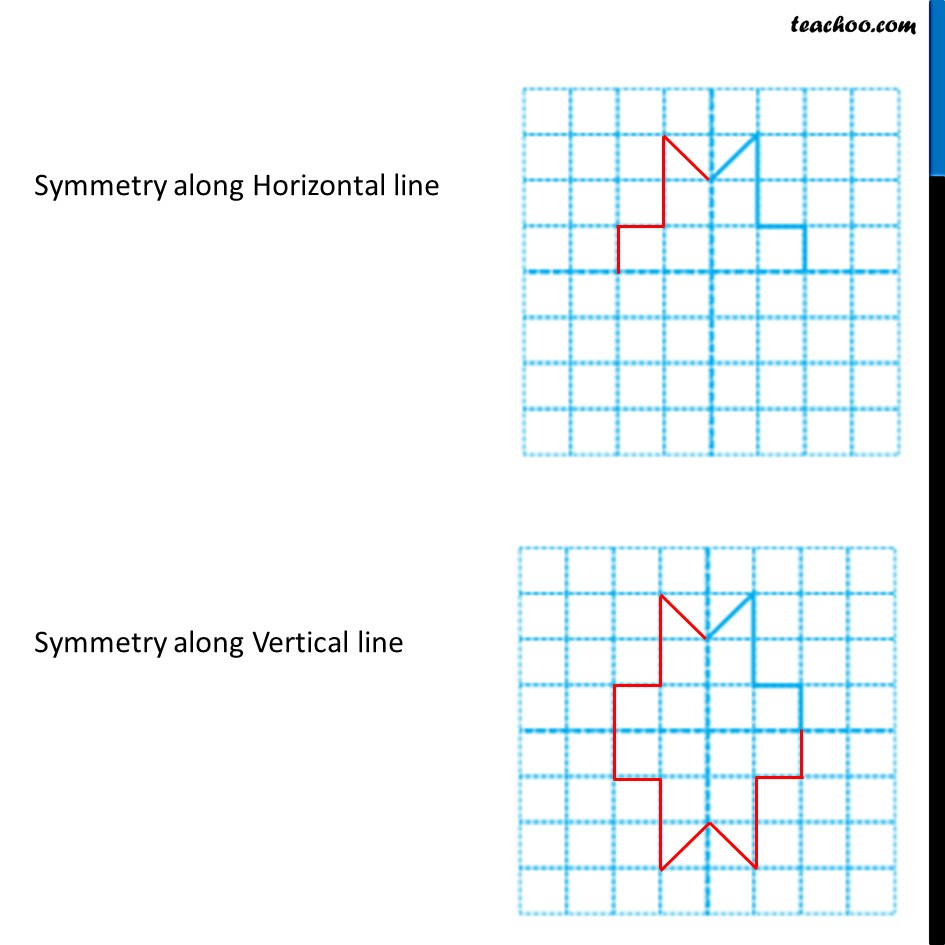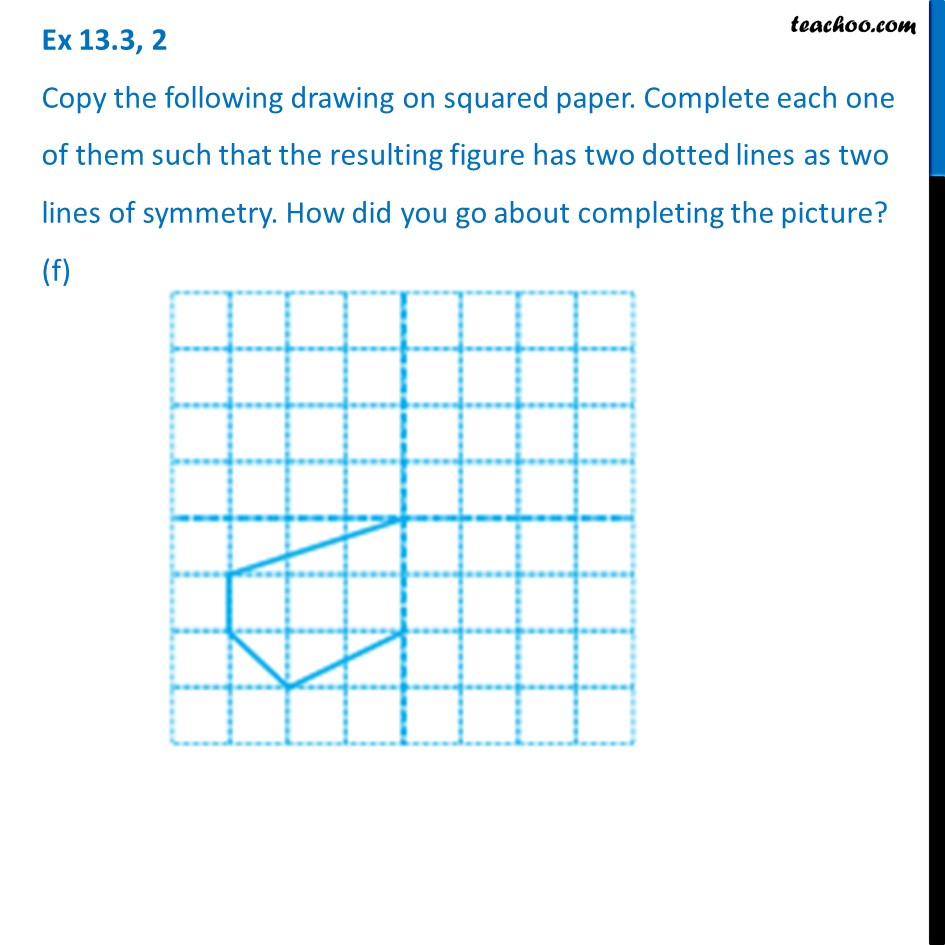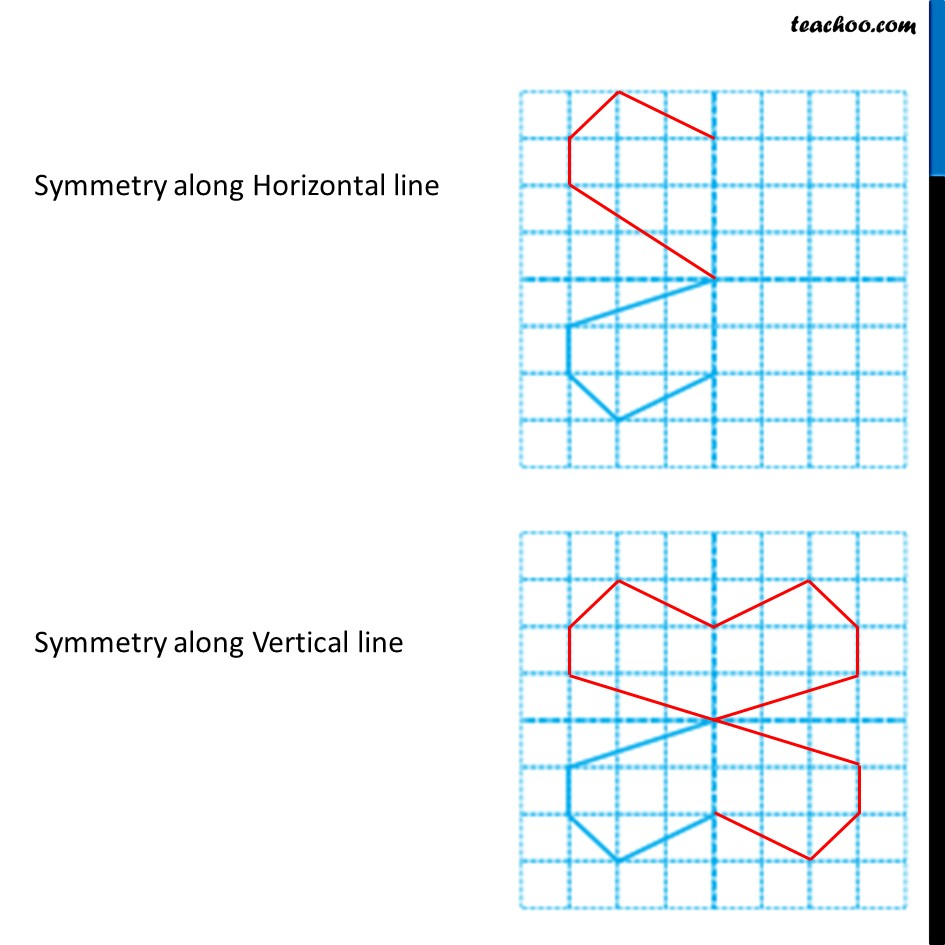1. Chapter 13 Class 6 Symmetry
2. Serial order wise
3. Ex 13.3

Transcript

Ex 13.3, 2 Copy the following drawing on squared paper. Complete each one of them such that the resulting figure has two dotted lines as two lines of symmetry. How did you go about completing the picture? (a) Symmetry along Horizontal line Symmetry along Vertical line Ex 13.3, 2 Copy the following drawing on squared paper. Complete each one of them such that the resulting figure has two dotted lines as two lines of symmetry. How did you go about completing the picture? (b) Symmetry along Horizontal line Symmetry along Vertical line Ex 13.3, 2 Copy the following drawing on squared paper. Complete each one of them such that the resulting figure has two dotted lines as two lines of symmetry. How did you go about completing the picture? (c) Symmetry along Horizontal line Symmetry along Vertical line Ex 13.3, 2 Copy the following drawing on squared paper. Complete each one of them such that the resulting figure has two dotted lines as two lines of symmetry. How did you go about completing the picture? (d) Symmetry along Horizontal line Symmetry along Vertical line Ex 13.3, 2 Copy the following drawing on squared paper. Complete each one of them such that the resulting figure has two dotted lines as two lines of symmetry. How did you go about completing the picture? (e) Symmetry along Horizontal line Symmetry along Vertical line Ex 13.3, 2 Copy the following drawing on squared paper. Complete each one of them such that the resulting figure has two dotted lines as two lines of symmetry. How did you go about completing the picture? (f) Symmetry along Horizontal line Symmetry along Vertical line

Ex 13.3

Chapter 13 Class 6 Symmetry
Serial order wise

About the AuthorDavneet Singh
Davneet Singh is a graduate from Indian Institute of Technology, Kanpur. He has been teaching from the past 10 years. He provides courses for Maths and Science at Teachoo.